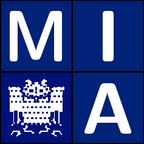Welcome to the homepage of the lecture

Wavelets and Sparsity

Summer Term 2012

Wavelets and Sparsity

Lecturers: Dr. Simon Setzer and Laurent Hoeltgen

Office hours: TBA

Summer Term 2012

Lectures (3h) with exercises (1h)
(6 credit points)

Time and Location: Tuesday 12-14 c.t. and Thursday 14-16 c.t., Building E1.3, Lecture Hall 001

First lecture: Tuesday, April 17, 2012

Exercises: Every second Thursday instead of a lecture, Laurent Hoeltgen will present the solution to the exercises.

Don't forget to schedule an appointment for your oral exam.

The wavelet transform allows us to represent data in a more suitable "basis". The resulting wavelet coefficients yield interesting ways to analyze and manipulate the data, e.g., compression (JPEG2000), multiscale analysis of seismic data, image denoising and inpainting. A central theme is that the given data is efficiently encoded in only relatively few wavelet coefficents ("sparsity"). We discuss not only the basic theory of the continuous and discrete wavelet transform but also algorithms and applications in image processing.

Undergraduate knowledge in mathematics (e.g. ''Mathematik für Informatiker I-III''). The lectures will be given in English.

DateTopicHomework
17.04. Introduction
19.04. Mathematical preliminaries Homework 1
03.05. Fourier Kingdom Homework 2, cameraman.pgm, fourier_approximation.c, fourier_approximation_soln.c, plot of the solution (zoomed in)
15.05. Homework 3
24.05. Windowed Fourier Transform
31.05. The wavelet transform
05.06. Parseval's formula for the wavelet transform Homework 4
26.06. The wavelet transform (cont.) Homework 5,FWT_1d.c, IFWT_1d.c,signal256.txt, FWT_1d_solution.c, IFWT_1d_solution.c
10.07. Redundant representations (Introduction and frames)
10.07. Redundant representations (Ridgelets and curvelets) Homework 6, WS12/Homework6_2D_FWT.zip
26.07. Redundant representations (Analysis and synthesis approaches)
26.07. Redundant representations (Optimization theory for the synthesis approach)

Homework will be assigned bi-weekly. To qualify for the exam you need 50% of the points from these assignments.

There will be an oral or a written exam depending on the number of students.

The Registration for the lecture is currently open. Please register here.
Remember that you also have to register for the exam in the HISPOS system of the Saarland University

No textbook is required for this course. Examples of books giving background material and further reading are:

1. Sparse Image and Signal Processing: Wavelets, Curvelets, Morphological Diversity
J.-L. Starck, F. Murtagh and J. M. Fadili, Cambridge University Press, 2010

2. A wavelet tour of signal processing: the sparse way (second edition)
S. G. Mallat, Academic Press, 2009

3. Sparse and Redundant Representations

4. Ten lectures on wavelets
I. Daubechies, SIAM, 2006

5. Wavelets Theory and Application
A. K. Louis, P. Maaß and A. Rieder, J. Wiley & Sons, Inc., 1997

6. Fourier Analysis and Applications
C. Gasquet and P. Witomski, Springer, 1998

MIA Group